### The Anomalous Zeeman Effect

We compute the energy change due to a weak magnetic field using first order Perturbation Theory.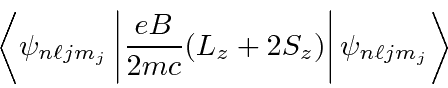Thepart is easy since we are in eigenstates of that operator.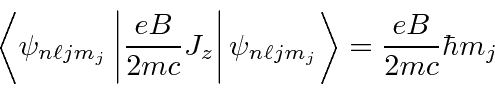The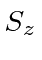is harder since we are not in eigenstates of that one. We need, but we don't know howacts on these. So, we must writein terms of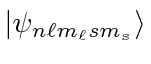.We already know how to write in terms of these states of definiteand.Let's do thestate first.For,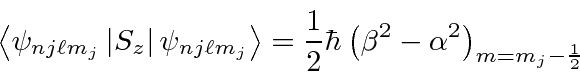We can combine the two formulas for.So adding this to the (easier) part above, we have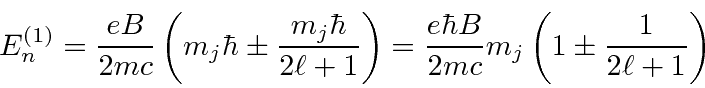for.

In summary then, we rewrite the fine structure shift.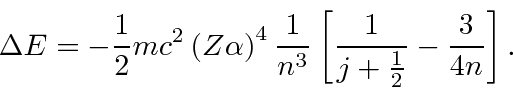To this we add the anomalous Zeeman effectJim Branson 2013-04-22